# Difference Between Multithreading vs Multiprocessing in Python

In this article, we will learn the what, why, and how of multithreading and multiprocessing in Python. Before we dive into the code, let us understand what these terms mean.

• A program is an executable file which consists of a set of instructions to perform some task and is usually stored on the disk of your computer.
• A process is what we call a program that has been loaded into memory along with all the resources it needs to operate. It has its own memory space.
• A thread is the unit of execution within a process. A process can have multiple threads running as a part of it, where each thread uses the process’s memory space and shares it with other threads.
• Multithreading is a technique where multiple threads are spawned by a process to do different tasks, at about the same time, just one after the other. This gives you the illusion that the threads are running in parallel, but they are actually run in a concurrent manner. In Python, the Global Interpreter Lock (GIL) prevents the threads from running simultaneously.
• Multiprocessing is a technique where parallelism in its truest form is achieved. Multiple processes are run across multiple CPU cores, which do not share the resources among them. Each process can have many threads running in its own memory space. In Python, each process has its own instance of Python interpreter doing the job of executing the instructions.

Now, let’s jump into the program where we try to execute two different types of functions: IO-bound and CPU-bound in six different ways. Inside the IO-bound function, we ask the CPU to sit idle and pass time whereas, inside the CPU-bound function, the CPU is going to be busy churning out a few numbers.

Requirements:

• A Windows computer (My machine has 6 cores).
• Python 3.x installed.
• Any text editor/IDE to write Python programs (I am using Sublime Text here).

Note: Below is the structure of our program, which will be common across all the six parts. In the place where it is mentioned # YOUR CODE SNIPPET HERE, replace it with the code snippet of each part as you go.

## Python3

 `import` `time, os` `from` `threading ``import` `Thread, current_thread` `from` `multiprocessing ``import` `Process, current_process`     `COUNT ``=` `200000000` `SLEEP ``=` `10`   `def` `io_bound(sec):`   `    ``pid ``=` `os.getpid()` `    ``threadName ``=` `current_thread().name` `    ``processName ``=` `current_process().name`   `    ``print``(f"{pid} ``*` `{processName} ``*` `{threadName} \` `        ``-``-``-``> Start sleeping...")` `    ``time.sleep(sec)` `    ``print``(f"{pid} ``*` `{processName} ``*` `{threadName} \` `        ``-``-``-``> Finished sleeping...")`   `def` `cpu_bound(n):`   `    ``pid ``=` `os.getpid()` `    ``threadName ``=` `current_thread().name` `    ``processName ``=` `current_process().name`   `    ``print``(f"{pid} ``*` `{processName} ``*` `{threadName} \` `        ``-``-``-``> Start counting...")`   `    ``while` `n>``0``:` `        ``n ``-``=` `1`   `    ``print``(f"{pid} ``*` `{processName} ``*` `{threadName} \` `        ``-``-``-``> Finished counting...")`   `if` `__name__``=``=``"__main__":` `    ``start ``=` `time.time()`   `    ``# YOUR CODE SNIPPET HERE`   `    ``end ``=` `time.time()` `    ``print``(``'Time taken in seconds -'``, end ``-` `start)`

Part 1: Running IO-bound task twice, one after the other…

## Python3

 `# Code snippet for Part 1` `io_bound(SLEEP)` `io_bound(SLEEP)`

Here, we ask our CPU to execute the function io_bound(), which takes in an integer (10, here) as a parameter and asks the CPU to sleep for that many seconds. This execution takes a total of 20 seconds, as each function execution takes 10 seconds to complete. Note that, it is the same MainProcess that calls our function twice, one after the other, using its default thread, MainThread.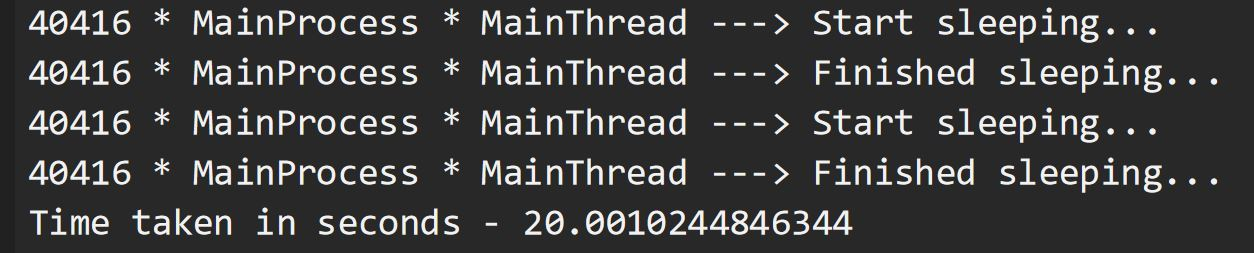## Python3

 `# Code snippet for Part 2` `t1 ``=` `Thread(target ``=` `io_bound, args ``=``(SLEEP, ))` `t2 ``=` `Thread(target ``=` `io_bound, args ``=``(SLEEP, ))` `t1.start()` `t2.start()` `t1.join()` `t2.join()`

Here, let’s use threading in Python to speed up the execution of the functions. The threads Thread-1 and Thread-2 are started by our MainProcess, each of which calls our function, at almost the same time. Both the threads complete their job of sleeping for 10 seconds, concurrently. This reduced the total execution time of our whole program by a significant 50%. Hence, multithreading is the go-to solution for executing tasks wherein the idle time of our CPU can be utilized to perform other tasks. Hence, saving time by making use of the wait time.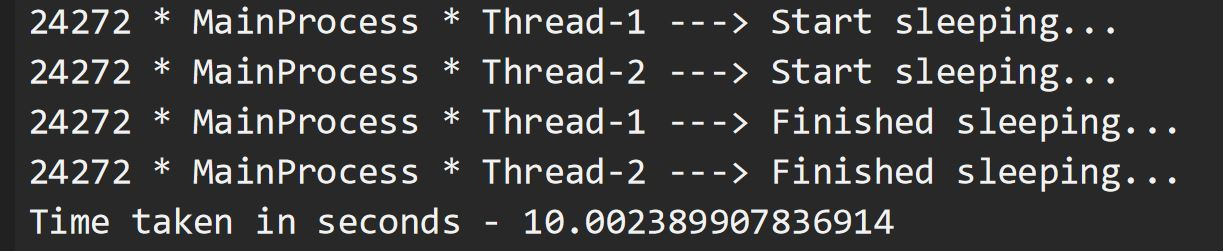Part 3: Running CPU-bound task twice, one after the other…

## Python3

 `# Code snippet for Part 3` `cpu_bound(COUNT)` `cpu_bound(COUNT)`

Here, we shall call our function cpu_bound(), which takes in a big number (200000000, here) as a parameter and decrements it at each step until it is zero. Our CPU is asked to do the countdown at each function call, which roughly takes around 12 seconds (this number might differ on your machine). Hence, the execution of the whole program took me about 26 seconds to complete. Note that it is once again our MainProcess calling the function twice, one after the other, in its default thread, MainThread.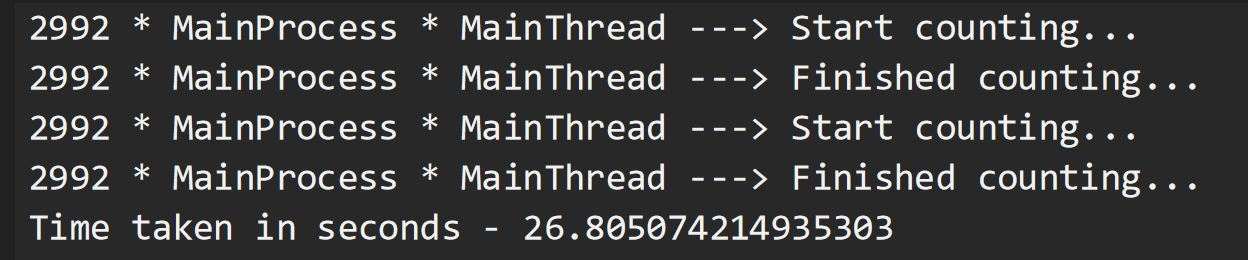## Python3

 `# Code snippet for Part 4` `t1 ``=` `Thread(target ``=` `cpu_bound, args ``=``(COUNT, ))` `t2 ``=` `Thread(target ``=` `cpu_bound, args ``=``(COUNT, ))` `t1.start()` `t2.start()` `t1.join()` `t2.join()`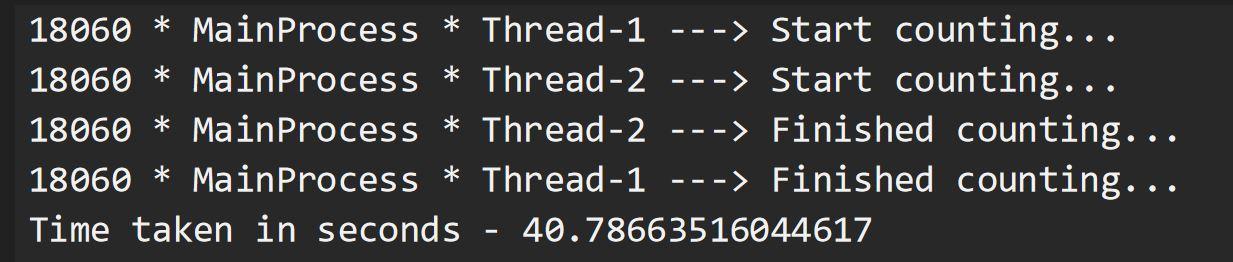Part 5: So, does splitting the tasks as separate processes work?

## Python3

 `# Code snippet for Part 5` `p1 ``=` `Process(target ``=` `cpu_bound, args ``=``(COUNT, ))` `p2 ``=` `Process(target ``=` `cpu_bound, args ``=``(COUNT, ))` `p1.start()` `p2.start()` `p1.join()` `p2.join()`

Let’s cut to the chase. Multiprocessing is the answer. Here the MainProcess spins up two subprocesses, having different PIDs, each of which does the job of decreasing the number to zero. Each process runs in parallel, making use of separate CPU core and its own instance of the Python interpreter, therefore the whole program execution took only 12 seconds. Note that the output might be printed in unordered fashion as the processes are independent of each other. Each process executes the function in its own default thread, MainThread. Open your Task Manager during the execution of your program. You can see 3 instances of the Python interpreter, one each for MainProcess, Process-1, and Process-2. You can also see that during the program execution, the Power Usage of the two subprocesses is “Very High”, as the task they are performing is actually taking a toll on their own CPU cores, as shown by the spikes in the CPU Performance graph.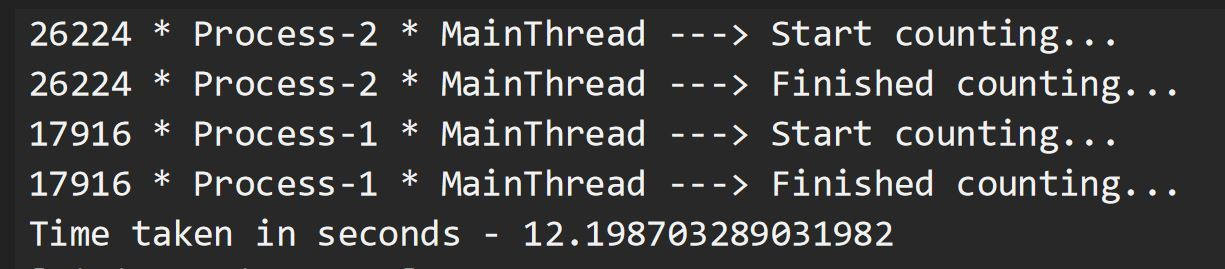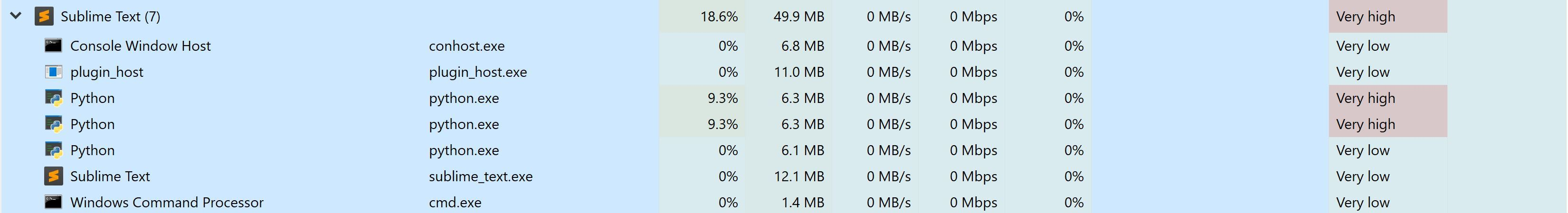Part 6: Hey, lets use multiprocessing for our IO-bound tasks…

## Python3

 `# Code snippet for Part 6` `p1 ``=` `Process(target ``=` `io_bound, args ``=``(SLEEP, ))` `p2 ``=` `Process(target ``=` `io_bound, args ``=``(SLEEP, ))` `p1.start()` `p2.start()` `p1.join()` `p2.join()`

Now that we got a fair idea about multiprocessing helping us achieve parallelism, we shall try to use this technique for running our IO-bound tasks. We do observe that the results are extraordinary, just as in the case of multithreading. Since the processes Process-1 and Process-2 are performing the task of asking their own CPU core to sit idle for a few seconds, we don’t find high Power Usage. But the creation of processes itself is a CPU heavy task and requires more time than the creation of threads. Also, processes require more resources than threads. Hence, it is always better to have multiprocessing as the second option for IO-bound tasks, with multithreading being the first.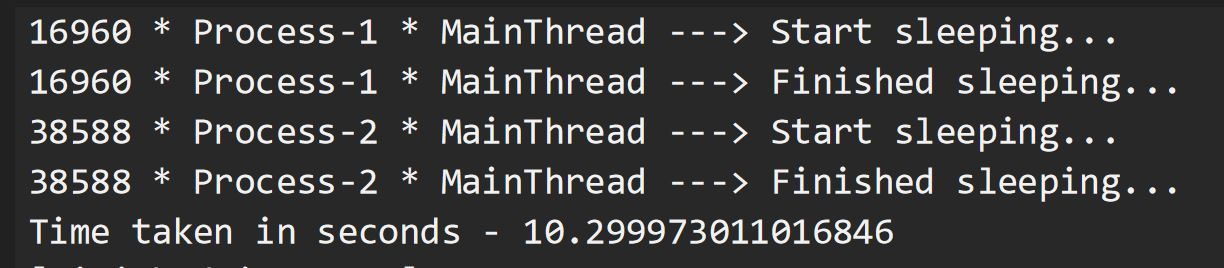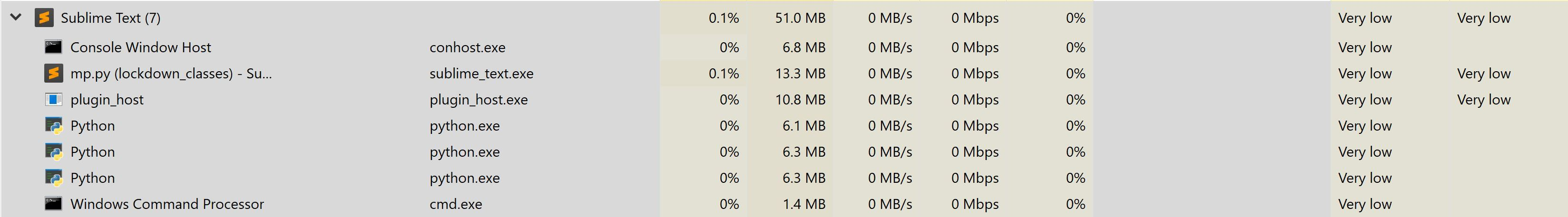Well, that was quite a ride. We saw six different approaches to perform a task, that roughly took about 10 seconds, based on whether the task is light or heavy on the CPU.